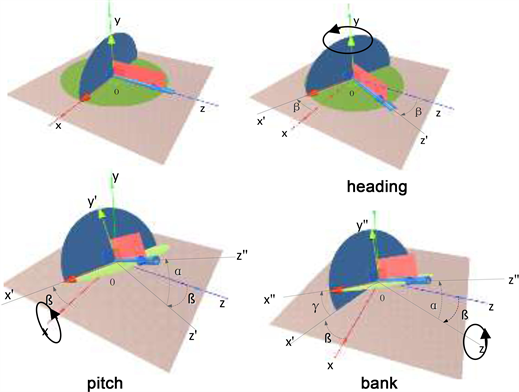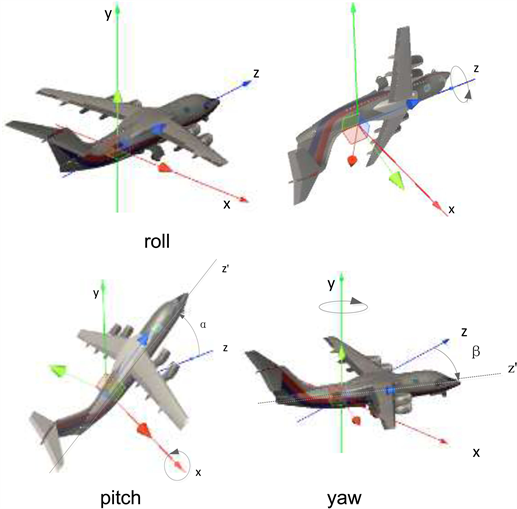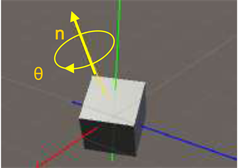# Unity 3D中欧拉角与四元数关系的研究Study on the Relationship between Euler An-gle and Quaternion in Unity 3D

• 全文下载: PDF(686KB)    PP.890-895   DOI: 10.12677/CSA.2019.95100
• 下载量: 352  浏览量: 693

In unity 3D game engine, the orientation of objects is stored in the form of quaternions, but the Euler angle is presented to the user on the interface. The user-set Euler angle is converted to quaternion first and finally to a new Euler angle, and each Euler angle can be expressed in two quaternions, which makes the problem very complicated. In this paper, two conventions of Euler angle and mathematical expressions of Euler angle and quaternion are given. By analyzing and studying the application of Euler angle and quaternion in unity 3D game engine, the transformation relationship between them is explained in detail.

1. 引言

2. 欧拉角和四元数的概念

2.1. 欧拉角的概念

“roll-pitch-yaw”约定只考虑惯性坐标系，旋转的顺序为“roll-pitch-yaw”，即：z → x → y，与heading-pitch-bank (y → x → z)约定正好相反，如图2所示。

2.2. 四元数的概念Figure 2. Roll-pitch-yaw

3. 欧拉角与四元数的转换

3.1. 从欧拉角转换到四元数

${q}_{h}=\left[\begin{array}{c}\mathrm{cos}\left({\theta }_{h}/2\right)\\ \left(\begin{array}{c}0\\ -\mathrm{sin}\left({\theta }_{h}/2\right)\\ 0\end{array}\right)\end{array}\right],\text{\hspace{0.17em}}\text{\hspace{0.17em}}{q}_{p}=\left[\begin{array}{c}\mathrm{cos}\left({\theta }_{p}/2\right)\\ \left(\begin{array}{c}-\mathrm{sin}\left({\theta }_{p}/2\right)\\ 0\\ 0\end{array}\right)\end{array}\right],\text{\hspace{0.17em}}\text{\hspace{0.17em}}{q}_{b}=\left[\begin{array}{c}\mathrm{cos}\left({\theta }_{b}/2\right)\\ \left(\begin{array}{c}0\\ 0\\ -\mathrm{sin}\left({\theta }_{b}/2\right)\end{array}\right)\end{array}\right]$

${q}_{h},{q}_{p},{q}_{b}$，是三次旋转，最终需要形成一个四元数q，将三者直接相乘即可，即 $q={q}_{h}{q}_{p}{q}_{b}$，矩阵相乘的顺序采用“heading-pitch-bank”约定。

$q={q}_{h}{q}_{p}{q}_{b}=\left[\begin{array}{c}\mathrm{cos}\left({\theta }_{h}/2\right)\mathrm{cos}\left({\theta }_{p}/2\right)\mathrm{cos}\left({\theta }_{b}/2\right)+\mathrm{sin}\left({\theta }_{h}/2\right)\mathrm{sin}\left({\theta }_{p}/2\right)\mathrm{sin}\left({\theta }_{b}/2\right)\\ \left(\begin{array}{c}-\mathrm{cos}\left({\theta }_{h}/2\right)\mathrm{sin}\left({\theta }_{p}/2\right)\mathrm{cos}\left({\theta }_{b}/2\right)-\mathrm{sin}\left({\theta }_{h}/2\right)\mathrm{cos}\left({\theta }_{p}/2\right)\mathrm{sin}\left({\theta }_{b}/2\right)\\ \mathrm{cos}\left({\theta }_{h}/2\right)\mathrm{sin}\left({\theta }_{p}/2\right)\mathrm{sin}\left({\theta }_{b}/2\right)-\mathrm{sin}\left({\theta }_{h}/2\right)\mathrm{cos}\left({\theta }_{p}/2\right)\mathrm{cos}\left({\theta }_{b}/2\right)\\ \mathrm{sin}\left({\theta }_{h}/2\right)\mathrm{sin}\left({\theta }_{p}/2\right)\mathrm{cos}\left({\theta }_{b}/2\right)-\mathrm{cos}\left({\theta }_{h}/2\right)\mathrm{cos}\left({\theta }_{p}/2\right)\mathrm{sin}\left({\theta }_{b}/2\right)\end{array}\right)\end{array}\right]$ (1)

3.2. 从四元数转换到欧拉角

${\theta }_{p}=\text{asin}\left(-2\left(yz+wx\right)\right)$

${\theta }_{h}=\left\{\begin{array}{l}\text{atan}2\left(xz-wy,1/2-{x}^{2}-{y}^{2}\right)\text{\hspace{0.17em}}\text{\hspace{0.17em}}\text{\hspace{0.17em}}若\mathrm{cos}p\ne 0\\ \text{atan}2\left(-xz-wy,1/2-{y}^{2}-{z}^{2}\right)\text{\hspace{0.17em}}\text{\hspace{0.17em}}\text{\hspace{0.17em}}\text{\hspace{0.17em}}其他\end{array}$ (2)

${\theta }_{b}=\left\{\begin{array}{l}\text{atan}2\left(xy-wz,1/2-{x}^{2}-{z}^{2}\right)\text{\hspace{0.17em}}\text{\hspace{0.17em}}\text{\hspace{0.17em}}\text{\hspace{0.17em}}若\mathrm{cos}p\ne 0\\ 0\text{\hspace{0.17em}}\text{\hspace{0.17em}}\text{\hspace{0.17em}}\text{\hspace{0.17em}}\text{\hspace{0.17em}}\text{\hspace{0.17em}}\text{\hspace{0.17em}}\text{\hspace{0.17em}}\text{\hspace{0.17em}}\text{\hspace{0.17em}}\text{\hspace{0.17em}}\text{\hspace{0.17em}}\text{\hspace{0.17em}}\text{\hspace{0.17em}}\text{\hspace{0.17em}}\text{\hspace{0.17em}}\text{\hspace{0.17em}}\text{\hspace{0.17em}}\text{\hspace{0.17em}}\text{\hspace{0.17em}}\text{\hspace{0.17em}}\text{\hspace{0.17em}}\text{\hspace{0.17em}}\text{\hspace{0.17em}}\text{\hspace{0.17em}}\text{\hspace{0.17em}}\text{\hspace{0.17em}}\text{\hspace{0.17em}}\text{\hspace{0.17em}}\text{\hspace{0.17em}}\text{\hspace{0.17em}}\text{\hspace{0.17em}}\text{\hspace{0.17em}}\text{\hspace{0.17em}}\text{\hspace{0.17em}}\text{\hspace{0.17em}}\text{\hspace{0.17em}}\text{\hspace{0.17em}}\text{\hspace{0.17em}}\text{\hspace{0.17em}}\text{\hspace{0.17em}}\text{\hspace{0.17em}}\text{\hspace{0.17em}}其他\end{array}$

4. 在Unity 3D中欧拉角和四元数的应用Figure 3. Determination of object orientation by angular pair

$q=\left(x,y,z,w\right)=\left({n}_{x}\mathrm{sin}\left(\theta /2\right),{n}_{y}\mathrm{sin}\left(\theta /2\right),{n}_{z}\mathrm{sin}\left(\theta /2\right),\mathrm{cos}\left(\theta /2\right)\right)$

Quaternion.identity

$q=\left(0\mathrm{sin}\left(360/2\right),1\mathrm{sin}\left(360/2\right),0\mathrm{sin}\left(360/2\right),\mathrm{cos}\left(360/2\right)\right)=\left(0,0,0,-1\right)$Table 1. Initial Euler angle, quaternion and final Euler angle

5. 分析及总结

  庞钦存. 四元数在游戏引擎Unity3D中的应用[J]. 产业与科技论坛, 2015, 14(17): 63-64.  李志伟, 李克昭, 赵磊杰, 王云凯, 梁晓庆. 基于单位四元数的任意旋转角度的三维坐标转换[J]. 大地测量与地球动力学, 2017, 37(1): 81-85.  鲁丹丹, 翟永翠, 周玉芳. 基于四元数的DR技术[J]. 指挥控制与仿真, 2019(2): 98-103.  张帆, 曹喜滨, 邹经湘. 一种新的全角度四元数与欧拉角的转换算法[J]. 南京理工大学学报(自然科学版), 2002(4): 376-380.  王彤, 马建仓, 秦涛, 柏会宁. 基于旋转四元数的姿态解算算法[J]. 弹箭与制导学报, 2014, 34(3): 15-16+20.